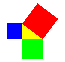#The Pythagorean Theorem

Math Lair Home > Topics > The Pythagorean TheoremThe Pythagorean Theorem states that the area of the square of the hypotenuse of a right-angled triangle is equal to the areas of the squares of the other two sides. If we let c represent the length of the hypotenuse, and a and b the two other sides (legs) of that triangle, then we get the famous formula a² + b² = c². While this relationship was known before the time of Pythagoras, it was either Pythagoras or one of his followers who proved the relationship.

There are many ways to prove the Pythagorean Theorem; Elisha Loomis, in The Pythagorean Proposition, compiled 370 different proofs, and asserted that there is no limit to the number of possible proofs. The book lists the proof given by Euclid in the Elements, proofs by Thabit ibn Qurra, Bhaskara, Huygens, Leibniz, Legendre, former United States President James Garfield, and several teenagers. Interestingly enough, it is not known which proof was discovered by Pythagoras or his followers.

The Guinness Book of World Records lists the Pythagorean theorem as being the world's most-proved theorem. View some of these. There's a nice, interactive Java-based proof at www.cut-the-knot.com. It's quite good. You can also view the proof in the Elements as given by Euclid.

Here is a simple, not particularly rigourous proof of the Pythagorean Theorem: Let's look at the square on the hypotenuse first. Its area, of course, is c². We could place four of our right triangles in the square, the hypotenuse of each one on a side of a square, like so:Notice that there is a square in the middle. You can check that each of its sides is the difference between the lengths of the two legs. We could calculate the area of each of the four triangles and the square, and show that this area adds up to a² + b². There is, however, a more elegant way to prove the theorem. We could rearrange the four triangles and the square like so:Notice that the black line in the picture divides the rearranged shape into two squares. The first square has a side length equal to the larger of the two leg lengths, and the second square has a side length equal to the smaller. Therefore, the total area of the two squares is equal to a² + b². Therefore, a² + b² = c². That concludes the proof.

The ancient Egyptians knew that you could make a right triangle of side lengths 3, 4, and 5. The Babylonians, while they never attempted to prove this relation, had experimentally observed this relationship and had found many right triangles with integer side lengths, including ones with sides as long as:

• 3367, 3456, and 4825
• 4800, 4601, and 6649
• 6480, 4961, and 8161.

Sets of integers that can form the sides of a right triangle, like the ones above, are known as Pythagorean triples. It turns out that there are an infinite number of them.